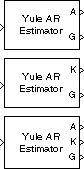# Yule-Walker AR Estimator

Compute estimate of autoregressive (AR) model parameters using Yule-Walker method

•Libraries:
DSP System Toolbox / Estimation / Parametric Estimation

## Description

The Yule-Walker AR Estimator block uses the Yule-Walker AR method, also called the autocorrelation method, to fit an autoregressive (AR) model to the windowed input data by minimizing the forward prediction error in the least squares sense. This process results in the Yule-Walker equations, which the block solves using the Levinson-Durbin recursion. The block outputs are always nonsingular.

## Ports

### Input

expand all

Specify the input data as a vector or a matrix. The block assumes the input data to be the output of an AR system driven by white noise.

When you specify a matrix, the block treats each column of the matrix as a channel. If the input is a row vector of length N, then the block treats the input as having N different channels. If the input is an unoriented vector, the block treats the input as a single channel.

#### Dependency

To use an input that is a row vector, set Output(s) to `A`.

Data Types: `single` | `double`

### Output

expand all

Normalized estimate of the AR model polynomial coefficients A(z), returned as a vector or a matrix. If the output is a vector, then it is of length p+1 in descending powers of z. If the output is a matrix, then each column of the matrix is of length p+1 and contains the normalized estimate of AR model coefficients in descending powers of z.

The block computes the estimate of these coefficients independently for each successive input frame.

`$H\left(z\right)=\frac{\sqrt{G}}{A\left(z\right)}=\frac{\sqrt{G}}{1+a\left(2\right){z}^{-1}+\dots +a\left(p+1\right){z}^{-p}}$`

where,

• H(z) –– Transfer function of the estimated AR model

• G –– Square of the model gain

• A(z) –– Polynomial coefficients of the AR model

#### Dependency

To enable this port, set the Output(s) parameter to `A` or ```A and K```.

Data Types: `single` | `double`

Reflection coefficients (which are a secondary result of the Levinson recursion), returned as a column vector, an unoriented vector of length p, or a matrix of size p-by-N, where N is the number of input channels. For each input channel, port K outputs a length-p column whose elements are the AR model reflection coefficients.

#### Dependency

To enable this port, set the Output(s) parameter to `K` or ```A and K```.

To output reflection coefficients K, specify the input to be a row vector, unoriented vector, or a matrix.

Data Types: `single` | `double`

Square of the gain of the estimated AR model for each input channel, returned as a scalar or a vector of length N, where N is the number of input channels. The port G outputs a scalar for each channel.

Here is the equation for the transfer function of the estimated AR model which shows the gain and the polynomial coefficients of the model:

`$H\left(z\right)=\frac{\sqrt{G}}{A\left(z\right)}=\frac{\sqrt{G}}{1+a\left(2\right){z}^{-1}+\dots +a\left(p+1\right){z}^{-p}}$`

where,

• H(z) –– Transfer function of the estimated AR model

• G –– Square of the model gain

• A(z) –– Polynomial coefficients of the AR model

Data Types: `single` | `double`

## Parameters

expand all

Specify whether the block outputs model coefficients (`A`), reflection coefficients (`K`), or both (`A and K`).

When you select the Inherit estimation order from input dimensions parameter, the block sets the order p of the all-pole model to a value that is one less than the length of each input channel. Otherwise, the order is the value you specify in the Estimation order parameter.

Specify the order of the AR model p as a nonnegative integer.

To guarantee a valid output, you must set the Estimation order parameter to be a scalar less than or equal to half the input channel length.

#### Dependencies

To enable this parameter, clear the Inherit estimation order from input dimensions parameter.

## Block Characteristics

 Data Types `double` | `single` Multidimensional Signals `No` Variable-Size Signals `No`